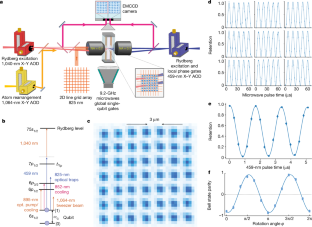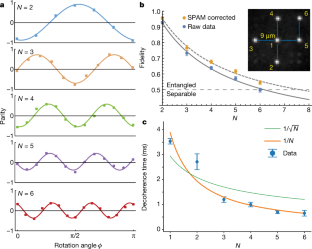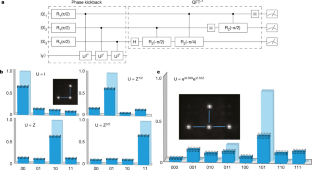Thank you for visiting nature.com. You are using a browser version with limited support for CSS. To obtain the best experience, we recommend you use a more up to date browser (or turn off compatibility mode in Internet Explorer). In the meantime, to ensure continued support, we are displaying the site without styles and JavaScript.

# Multi-qubit entanglement and algorithms on a neutral-atom quantum computer

## Abstract

Gate-model quantum computers promise to solve currently intractable computational problems if they can be operated at scale with long coherence times and high-fidelity logic. Neutral-atom hyperfine qubits provide inherent scalability owing to their identical characteristics, long coherence times and ability to be trapped in dense, multidimensional arrays1. Combined with the strong entangling interactions provided by Rydberg states2,3,4, all the necessary characteristics for quantum computation are available. Here we demonstrate several quantum algorithms on a programmable gate-model neutral-atom quantum computer in an architecture based on individual addressing of single atoms with tightly focused optical beams scanned across a two-dimensional array of qubits. Preparation of entangled Greenberger–Horne–Zeilinger (GHZ) states5 with up to six qubits, quantum phase estimation for a chemistry problem6 and the quantum approximate optimization algorithm (QAOA)7 for the maximum cut (MaxCut) graph problem are demonstrated. These results highlight the emergent capability of neutral-atom qubit arrays for universal, programmable quantum computation, as well as preparation of non-classical states of use for quantum-enhanced sensing.

This is a preview of subscription content, access via your institution

## Access options

\$32.00

All prices are NET prices.Fig. 1: Experimental quantum computing platform.Fig. 2: Preparation of GHZ states.Fig. 3: Quantum phase estimation using three and four qubits.Fig. 4: QAOA algorithm for solving MaxCut.

## Data availability

The data presented here are available from the corresponding author on reasonable request.

## References

1. Saffman, M., Walker, T. G. & Mølmer, K. Quantum information with Rydberg atoms. Rev. Mod. Phys. 82, 2313–2363 (2010).

2. Jaksch, D. et al. Fast quantum gates for neutral atoms. Phys. Rev. Lett. 85, 2208–2211 (2000).

3. Gaëtan, A. et al. Observation of collective excitation of two individual atoms in the Rydberg blockade regime. Nat. Phys. 5, 115–118 (2009).

4. Urban, E. et al. Observation of Rydberg blockade between two atoms. Nat. Phys. 5, 110–114 (2009).

5. Greenberger, D. M., Horne, M. A. & Zeilinger, A. in Bell’s Theorem, Quantum Theory and Conceptions of the Universe (ed. Kafatos, M.) 69–72 (Springer, 1989).

6. Aspuru-Guzik, A., Dutoi, A. D., Love, P. J. & Head-Gordon, M. Simulated quantum computation of molecular energies. Science 309, 1704–1707 (2005).

7. Farhi, E., Goldstone, J. & Gutmann, S. A quantum approximate optimization algorithm. Preprint at https://arxiv.org/abs/1411.4028 (2014).

8. Martinez, E. A. et al. Real-time dynamics of lattice gauge theories with a few-qubit quantum computer. Nature 534, 516–519 (2016).

9. Figgatt, C. et al. Complete 3-qubit Grover search on a programmable quantum computer. Nat. Commun. 8, 1918 (2017).

10. DiCarlo, L. et al. Demonstration of two-qubit algorithms with a superconducting quantum processor. Nature 460, 240–244 (2009).

11. Harrigan, M. P. et al. Quantum approximate optimization of non-planar graph problems on a planar superconducting processor. Nat. Phys. 17, 332–336 (2021).

12. Watson, T. F. et al. A programmable two-qubit quantum processor in silicon. Nature 555, 633–637 (2018).

13. Zhou, X.-Q., Kalasuwan, P., Ralph, T. C. & O’Brien, J. L. Calculating unknown eigenvalues with a quantum algorithm. Nat. Photonics 7, 223–228 (2013).

14. Scholl, P. et al. Quantum simulation of 2D antiferromagnets with hundreds of Rydberg atoms. Nature 595, 233–238 (2021).

15. Ebadi, S. et al. Quantum phases of matter on a 256-atom programmable quantum simulator. Nature 595, 227–232 (2021).

16. Hauke, P., Cucchietti, F. M., Tagliacozzo, L., Deutsch, I. & Lewenstein, M. Can one trust quantum simulators? Rep. Prog. Phys. 75, 082401 (2012).

17. Xia, T. et al. Randomized benchmarking of single-qubit gates in a 2D array of neutral-atom qubits. Phys. Rev. Lett. 114, 100503 (2015).

18. Wang, Y., Kumar, A., Wu, T.-Y. & Weiss, D. S. Single-qubit gates based on targeted phase shifts in a 3D neutral atom array. Science 352, 1562–1565 (2016).

19. Graham, T. et al. Rydberg mediated entanglement in a two-dimensional neutral atom qubit array. Phys. Rev. Lett. 123, 230501 (2019).

20. Barredo, D., de Leséléuc, S., Lienhard, V., Lahaye, T. & Browaeys, A. An atom-by-atom assembler of defect-free arbitrary two-dimensional atomic arrays. Science 354, 1021–1023 (2016).

21. Endres, M. et al. Atom-by-atom assembly of defect-free one-dimensional cold atom arrays. Science 354, 1024–1027 (2016).

22. Kim, H. et al. In situ single-atom array synthesis using dynamic holographic optical tweezers. Nat. Commun. 7, 13317 (2016).

23. Gisin, N. & Bechmann-Pasquinucci, H. Bell inequality, Bell states and maximally entangled states for n qubits. Phys. Lett. A 246, 1–6 (1998).

24. Song, C. et al. Generation of multicomponent atomic Schrödinger cat states of up to 20 qubits. Science 365, 574–577 (2019).

25. Pogorelov, I. et al. Compact ion-trap quantum computing demonstrator. PRX Quantum 2, 020343 (2021).

26. Omran, A. et al. Generation and manipulation of Schrödinger cat states in Rydberg atom arrays. Science 365, 570–574 (2019).

27. Wineland, D. J., Bollinger, J. J., Itano, W. M., Moore, F. L. & Heinzen, D. J. Spin squeezing and reduced quantum noise in spectroscopy. Phys. Rev. A 46, R6797–R6800 (1992).

28. Giovannetti, V., Lloyd, S. & Maccone, L. Quantum-enhanced measurements: beating the standard quantum limit. Science 306, 1330–1336 (2004).

29. Saffman, M. & Walker, T. G. Analysis of a quantum logic device based on dipole-dipole interactions of optically trapped Rydberg atoms. Phys. Rev. A 72, 022347 (2005).

30. Carr, A. W. & Saffman, M. Doubly magic optical trapping for Cs atom hyperfine clock transitions. Phys. Rev. Lett. 117, 150801 (2016).

31. Monz, T. et al. 14-qubit entanglement: creation and coherence. Phys. Rev. Lett. 106, 130506 (2011).

32. Abrams, D. S. & Lloyd, S. Quantum algorithm providing exponential speed increase for finding eigenvalues and eigenvectors. Phys. Rev. Lett. 83, 5162–5165 (1999).

33. Bravyi, S. B. & Kitaev, A. Y. Fermionic quantum computation. Ann. Phys. 298, 210–226 (2002).

34. Bravyi, S., Gambetta, J. M., Mezzacapo, A. & Temme, K. Tapering off qubits to simulate fermionic Hamiltonians. Preprint at https://arxiv.org/abs/1701.08213 (2017).

35. Kołos, W., Szalewicz,, K. & Monkhorst, H. J. New Born–Oppenheimer potential energy curve and vibrational energies for the electronic ground state of the hydrogen molecule. J. Chem. Phys. 84, 3278–3283 (1986).

36. Preskill, J. Quantum computing in the NISQ era and beyond. Quantum 2, 79 (2018).

37. Peruzzo, A. et al. A variational eigenvalue solver on a photonic quantum processor. Nat. Commun. 5, 4213 (2014).

38. Shor, P. W. Algorithms for quantum computation: discrete logarithms and factoring. In Proc. 35th Annual Symposium on Foundations of Computer Science 124–134 (IEEE, 1994).

39. Harrow, A. W., Hassidim,, A. & Lloyd, S. Quantum algorithm for linear systems of equations. Phys. Rev. Lett. 103, 150502 (2009).

40. O’Brien, T. E., Tarasinski, B. & Terhal, B. M. Quantum phase estimation of multiple eigenvalues for small-scale (noisy) experiments. New J. Phys. 21, 023022 (2019).

41. Endo, S., Cai, Z., Benjamin, S. C. & Yuan, X. Hybrid quantum-classical algorithms and quantum error mitigation. J. Phys. Soc. Jpn. 90, 032001 (2021).

42. Bluvstein, D. et al. A quantum processor based on coherent transport of entangled atom arrays. Nature https://doi.org/10.1038/s41586-022-04592-6 (2022).

43. Hsiao, Y.-F., Lin, Y.-J. & Chen, Y.-C. Λ-enhanced gray-molasses cooling of cesium atoms on the D2 line. Phys. Rev. A 98, 033419 (2018).

44. Gillen-Christandl, K., Gillen, G., Piotrowicz, M. J. & Saffman, M. Comparison of Gaussian and super Gaussian laser beams for addressing atomic qubits. Appl. Phys. B 122, 131 (2016).

45. Gullion, T., Baker, D. B. & Conradi, M. S. New, compensated Carr-Purcell sequences. J. Magn. Reson. 89, 479–484 (1990).

46. Kuhr, S. et al. Analysis of dephasing mechanisms in a standing-wave dipole trap. Phys. Rev. A 72, 023406 (2005).

47. Levine, H. et al. Parallel implementation of high-fidelity multiqubit gates with neutral atoms. Phys. Rev. Lett. 123, 170503 (2019).

48. Robicheaux, F., Graham, T. & Saffman, M. Photon-recoil and laser-focusing limits to Rydberg gate fidelity. Phys. Rev. A 103, 022424 (2021).

49. Saffman, M., Beterov, I. I., Dalal, A., Paez, E. J. & Sanders, B. C. Symmetric Rydberg controlled-Z gates with adiabatic pulses. Phys. Rev. A 101, 062309 (2020).

50. Zhang, S., Robicheaux, F. & Saffman, M. Magic wavelength optical traps for Rydberg atoms. Phys. Rev. A 84, 043408 (2011).

## Acknowledgements

This work was supported by DARPA ONISQ contract no. HR001120C0068, NSF award PHY-1720220, NSF award 2016136 for the QLCI for Hybrid Quantum Architectures and Networks, U.S. Department of Energy under award no. DE-SC0019465 and through Innovate UK’s Sustainable Innovation Fund Small Business Research Initiative (SBRI).

## Author information

Authors

### Contributions

T.M.G., Y.S., J.S., C.P., K.J., P.E., X.J., A.M., B.G., M.K., M.E., J.C., M.T.L., M.G., J.G., D.B., T.B., T.N. and M.S. contributed to the design and construction of the apparatus, including the classical control system. T.M.G., Y.S., J.S., K.J., L.P. and P.E. contributed to operating the apparatus and collecting and analysing the data. T.M.G., Y.S., C.C., E.D.D., C.P., L.P., O.C., N.S.B., B.R. and M.S. contributed to circuit design, optimization and simulation. The manuscript was written by T.M.G., Y.S., J.S., O.C., N.S.B., B.R. and M.S. T.M.G. and Y.S. contributed equally to this work. The project was supervised by T.N. and M.S. All authors discussed the results and contributed to the manuscript.

### Corresponding author

Correspondence to M. Saffman.

## Ethics declarations

### Competing interests

M.E., J.C., M.T.L., M.G., J.G., D.B., T.B., C.C., E.D.D., T.N. and M.S. are shareholders of ColdQuanta, Inc. O.C., N.S.B. and B.R. are employees of Riverlane, Ltd.

## Peer review

### Peer review information

Nature thanks Hannah Williams and the other, anonymous, reviewers for their contribution to the peer review of this work.

Publisher’s note Springer Nature remains neutral with regard to jurisdictional claims in published maps and institutional affiliations.

## Extended data figures and tables

### Extended Data Fig. 1 Optical module for generation of trapping light.

The light enters by means of a single-mode fibre that is collimated and then converted into a uniform intensity line with a diffractive optical element. The line is then divided into N lines by driving an AOD with N RF tones. This procedure is performed in two paths to create arrays of horizontal and vertical lines. The arrays are then combined on a polarizing beam splitter to create the grid of trap light, which is imaged onto the atoms.

### Extended Data Fig. 2 Characterization of optical trap array.

a, Geometry of the line array. b, Intensity landscape of traps for s = 3. c, Vibrational frequencies. The circles show the measured trap vibration frequencies of 19 kHz radial and 4 kHz axial. Plots used parameters d = 3 μm, s = 3, Ud = kB × 300 μK, Ta = 5 μK and λ = 825 nm. d, Plots of spatial localization. e, Map of measured variation in qubit frequency across the trap array, together with the measured shifts for the sites that were used relative to site q1. f, Ideal Gaussian beam intensity profile on a log scale.

### Extended Data Fig. 3 Rydberg laser stabilization.

a, Schematic of locking setup. b, Measured self-heterodyne spectrum of 918-nm laser with a 10-km fibre delay line.

### Extended Data Fig. 4 Calculated qubit coherence.

Calculated $${T}_{2}^{\ast }$$ as a function of atom temperature and magnetic field noise for 825-nm trap light and a bias magnetic field of 1.6 mT. The contours are labelled with the value of $${T}_{2}^{\ast }$$ in ms. The estimated magnetic noise on the basis of magnetometer measurements is σ < 20 mG and the average measured coherence time is $$\langle {T}_{2}^{\ast }\rangle =3.5{\rm{ms}},$$ which indicates an atomic temperature near 5 μK.

### Extended Data Fig. 5 Randomized benchmarking of single-qubit gates.

a, Characterization of SPAM errors in a 49-qubit array. The array-averaged SPAM error is 3.1%. b, $${{\rm{R}}}_{\varphi }^{({\rm{G}})}(\theta )$$ gate fidelity for Clifford gates. The array-averaged error per gate is 2.2 × 10−3. c, Characterization of SPAM errors for the six sites used in the main text. The average SPAM error is 2.5%. d, Fidelity of RZ(θ) and Rϕ(θ) gates. The average error per gate is 7.5 × 10−3.

### Extended Data Fig. 6 Analysis of one-qubit gate fidelity.

a, Amplitude of Rabi oscillation owing to shot-to-shot intensity noise for several values of the pulse area. b, Measured shot-to-shot variation of the 459-nm pulse power at a location after the AOD scanners in front of the vacuum cell. c, Simulated Ramsey signal with RZ(ϕ) gate (red dots) and fit to $${a}_{1}+{a}_{2}\,\cos ({a}_{3}\varphi ){{\rm{e}}}^{-\varphi /{a}_{4}}$$ (blue lines), which gives  = 46. The numerical simulation is integrated over the 3D atom and light distributions using λtrap = 825 nm, wline = 1 μm, d = 3 μm, Ud = 300 μK, w459 = 3 μm and Ta = 5 μK.

### Extended Data Fig. 7 CZ gate implementation and characterization by preparation of Bell states.

a, Symmetric CZ gate with phase-correction pulses. The gate in the dashed box is the uncorrected $${{\rm{C}}{\prime} }_{{\rm{Z}}}$$ that is composed of two Rydberg pulses that collectively drive both atoms. Pulses are detuned from resonance by Δ and have a single-atom resonant Rabi frequency of ΩR. The detuned, single-atom Rabi frequency is $$\Omega {\prime} =\sqrt{{\Omega }_{{\rm{R}}}^{2}+{\Delta }^{2}}.$$ The second pulse is driven with a phase ξ relative to the first pulse. The two phase-compensation pulses correct residual single-atom phases, ϕ01 and ϕ10, and are used to transform the gate into a canonical CZ. In principle, the compensation phases should be the same, but in practice, we find that better gate performance is achieved by allowing the phases to differ, which compensates for the lack of perfect balance between the Rydberg pulses at the two sites. The phase-compensation pulses can be applied before or after the Rydberg pulses. b, Two qubits spaced by d = 3 μm addressed with large beams of waist w = 7.5 μm focused halfway in between the qubits. At d = 3 μm, there is a strong blockade of B/2π = 1.03 GHz. Measured values were PBell = 0.475(0.01), parity amplitude C = 0.440(0.01) and fidelity FBell = 0.914(0.014). c, Two qubits spaced by d = 9 μm addressed with separate beams with waist w = 3 μm. At this spacing, the blockade is weak, B/2π = 3.0 MHz. Measured values were PBell = 0.483(0.009), parity amplitude C = 0.444(0.010) and fidelity FBell = 0.927(0.013). d, Characterization of CZ gate fidelity for five qubit pairs. Reported values are without SPAM correction and the average fidelity is 0.90.

## Supplementary information

### Supplementary Information

The supplementary information contains the complete circuit diagrams used for the GHZ state preparation, phase estimation and QAOA algorithms.

## Rights and permissions

Reprints and Permissions

• ### An architecture for quantum networking of neutral atom processors

• C. B. Young
• A. Safari
• M. Saffman

Applied Physics B (2022)